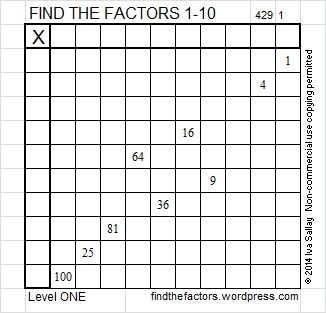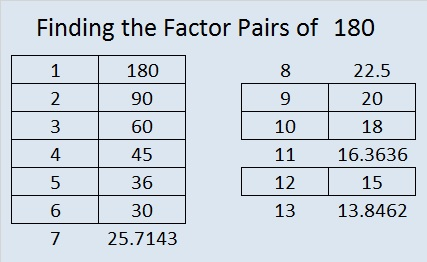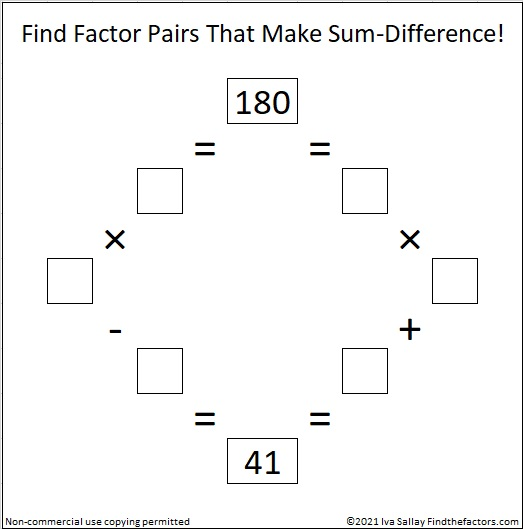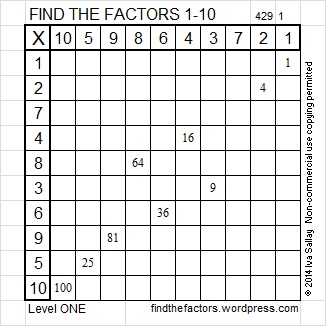# 180 and Level 1

Contents

### Today’s Puzzle:Excel file of puzzles and previous week’s factor solutions: 10 Factors 2014-07-21

### Factors of 180:

 180 is a composite number. Factor pairs: 180 = 1 x 180, 2 x 90, 3 x 60, 4 x 45, 5 x 36, 6 x 30, 9 x 20, 10 x 18, or 12 x 15 Factors of 180: 1, 2, 3, 4, 5, 6, 9, 10, 12, 15, 18, 20, 30, 36, 45, 60, 90, 180 Prime factorization: 180 = 2 x 2 x 3 x 3 x 5 which can be written 180 = (2^2) x (3^2) x 5### Sum-Difference Puzzle:

180 has nine factor pairs. One of the factor pairs adds up to 41, and another one subtracts to 41. If you can identify those two factor pairs, then you can solve this puzzle!### More about the Number 180:

There are 180 degrees in a triangle, and a straight angle is 180 degrees.

180 is the hypotenuse of a Pythagorean triple:
108-144-180, which is (3-4-5) times 36.

### Solution to This Week’s Puzzle:This site uses Akismet to reduce spam. Learn how your comment data is processed.